• Home
• SOLUTIONS
• PRODUCTS
• NEWS & Blogs

# Optical Gas Imaging Sensitivity StudyTIME TO READ

7 minsWRITTEN BY

Dr. Ram Hashmonaypublished

April 02, 2019CATEGORIES

## Introduction

The traditional method 21-based Leak Detection and Repair (LDAR) program quantifies leaks in volumetric concentration units (ppm v). Leak detection with Optical Gas Imaging (OGI) technology was initially considered as a non-quantifying technology, however recently capabilities of detecting leak’s mass flow rate were introduced using OGI technology. One of the major regulatory impediments for fully accepting the OGI technology as an alternative and superior LDAR practice, is the lack of rigorous understating of the technology’s envelope of sensitivity performance, compared to the volumetric concentrations provided by the traditional PID/FID-based “sniffers”. This extended abstract summarizes the tested sensitivity envelope of Opgal’s EyeCGas cooled OGI camera in various indoors and outdoors conditions. It also attempts to bring closure to the issue of understanding mass flow rate sensitivity in terms of volumetric concentrations.

## Envelope of Sensitivity Performance

### Indoor Laboratory Setting

Sensitivity of OGI technology for a specific compound is typically defined in terms of mass flow rate (grams per hour) of the leak. In a 2011 third party study[i], a contractor has tested and established that the mass flow detection limit of the EyeCGas cooled detector camera for methane was 0.35 g/hr at a temperature difference ΔT=2°C, and at distance of 2 meters from the leak (indoors laboratory settings, no wind).

This minimum detectable flow rate for methane in indoors laboratory settings can be extrapolated to other gases given the spectral absorption response in the camera’s filter region. For the OGI technology, it is a known practice to calculate the total absorption response of each compound relative to propane’s total absorption response, in the spectral range of the camera’s filter. The absorbance spectrum of methane and propane, collected for the same path integrated volumetric concentration and in the same conditions (1 ppm m, 25°C and 1 atmosphere), are shown in Figure 1 below. The transmittance of the EyeCGas filter is also shown.```Figure 1. EyeCGas standard filter transmittance along with the absorbance
spectra of methane and propane collected at 1 ppm m (@1 atm and 250°C).```

The volumetric absorption relative to propane (VARP) is calculated for each compound by ratio given in Equation 1: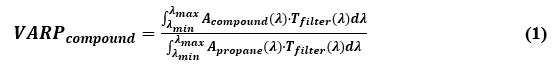where

Acompound(λ) – Absorbance value at each wavelength for the relevant compound.

Apropance(λ) – Absorbance value at each wavelength for propane.

Tfilter(λ) – Transmittance value at each wavelength for the camera’s filter.

λmin and λmax – The short and long edge wavelengths of the camera’s filter, respectively.

The mass flow detection limit (MFDLcompound) at ΔT=2°C in indoors laboratory settings is calculated for each compound from the measured MFDL for methane at ΔT=2°C in the test performed1 in 2011, MFDLmethane = 0.35 g/h, as shown in Equation 2: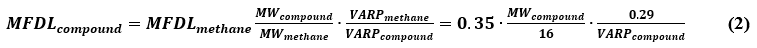where

MFDLmethane – Measured mass flow detection limit for methane at ΔT=2°C: 0.35 g/hr.

MWcompound – Molecular weight of compound in g/mol.

MWmethane – Molecular weight of methane: 16 g/mol.

VARPmethane – Methane’s coefficient of volumetric absorption relative to propane as calculated by Equation 1: 0.29.

VARPcompound – Compound’s coefficient of volumetric absorption relative to propane as calculated by Equation 1

Table 1 below summarizes the EyeCGas VARP coefficients and MFDL values for typical industrial compounds, as calculated by Equation 1 and 2 respectively.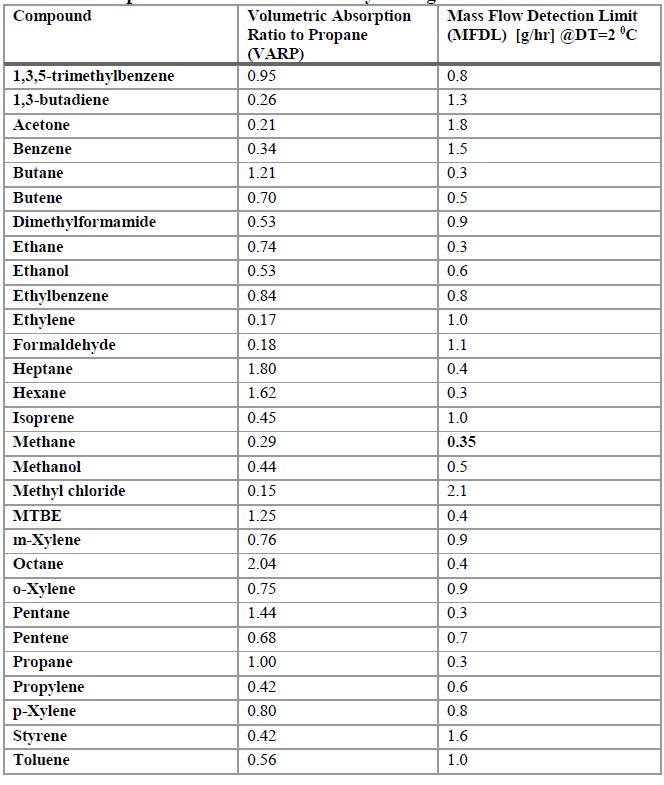```Table 1. Summary Table for EyeCGas VARP coefficients and MFDL values
for typical industrial compounds in indoors laboratory settings.```

### Outdoor “Real World” Settings

In a third-party outdoors study[ii], leaks were detected from longer distances and at various controlled cross wind speeds, to mimic real world scenarios. The envelope of sensitivity performance for methane (distances from the leak up to 12 meters and wind speeds up to 10 m/s) was calculated at ΔT≤2°C and summarized in Figure 2 below.

Figure 2. EyeCGas performance sensitivity envelope for pure methane leak, at various distances and cross wind speeds.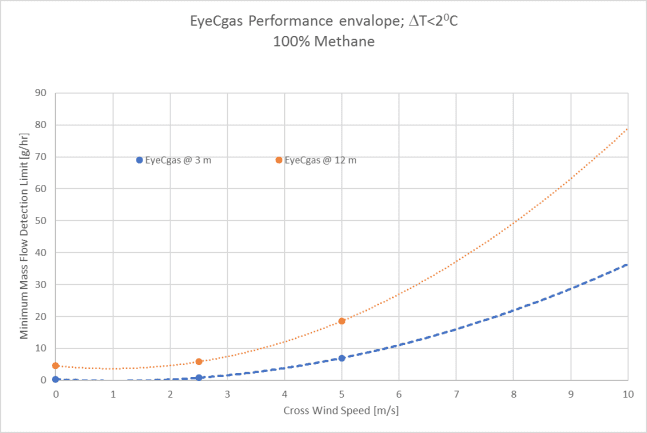```Figure 2. EyeCGas performance sensitivity envelope for pure
methane leak, at various distances and cross wind speeds.```

As can be seen in Figure 2, the EyeCGas camera could detect 0.34 g/hr of methane from 3 meters and no cross wind (very similar and slightly better than the results from the 2011 study). The study also demonstrates that sensitivity decreases with increased distance and wind speed. At 12 m distance and 5 m/s cross wind speed the camera could detect below 20 g/hr of methane (ΔT<2°C).

In Figure 3 below, a mixture of methane and benzene was tested in the same conditions as the pure methane.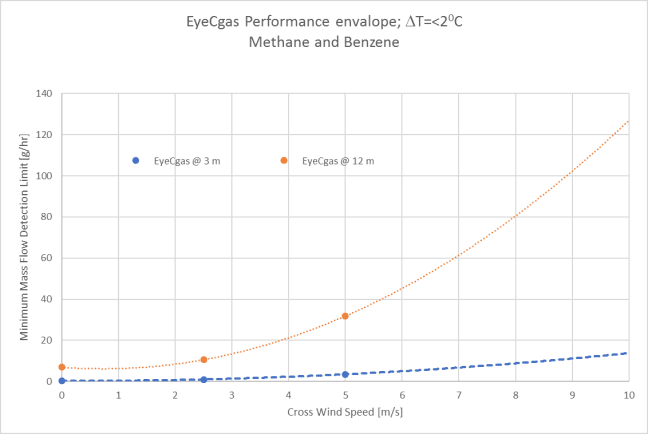```Figure 3. EyeCGas performance sensitivity envelope for a mixture of
benzene and methane leak, at various distances and cross wind speeds.```

As can be seen in Figure 3, the EyeCGas camera could detect 0.35 g/hr of the mixture from 3 meters and no cross wind (very similar to the pure methane in Figure 2). Also for this mixture sensitivity decreases with increased distance and wind speed. However, the general sensitivity for observation from 12 m, sensitivity is worse by almost a factor of 2. At 12 m distance and 5 m/s cross wind speed the camera could detect above 30 g/hr of the mixture of methane and benzene (ΔT<2°C).

### Mass Flow to Volumetric Concentration Conversion

Since the inception of LDAR programs in the 1980’s, it has been a great challenge to convert concentrations in the vicinity of the detected leak to mass emission/leak rate. Sensitivity of the OGI technology is typically defined and tested in mass leak rate units (g/hr). The inability to convert mass leak rate has been a long-time impediment for implementing OGI technology for LDAR programs. This section of the extended abstract attempts to map the volumetric concentration of an OGI detected (0.35 g/hr) plume. The mass flow rate detection limit in g/hr can be easily converted to volumetric flow rate in L/min using Equation 3 below: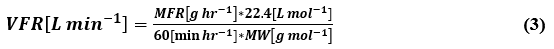where

VFR – Volumetric flow rate in liter per minute.
MFR – Mass flow rate in grams per hour.
MW – Molecular weight of compound in g/mol.

The molar volume of any gas is 22.4 liter per mole at standard temperature of 273°K and atmospheric pressure of 1 atmosphere. At 293°K (200°C) the molar volume is 24 L mol-1.

Typical flow rate of PID/FID-based “sniffers” is 1 L/min. Knowing the analyzer’s flow rate and assuming that the entire leak is captured by the analyzer, the upper bound volumetric concentration can be calculated for any given mass leak rate. The minimum detectable mass flow rate of an OGI camera is therefore may be converted to the maximum volumetric concentration that can be measured by a calibrated PID/FID-based analyzer. For example, using Eq. 3 one can calculate the maximum possible volumetric concentration in a vicinity of a methane leak of 0.35 g/hr (EyeCGas detection limit) to be in the range of 8000 ppm to 9000 ppm, depends of on atmospheric temperature and pressure (sea level, l0 – 25°C).

Recently, Opgal has performed a preliminary study in indoors laboratory settings, to measure the volumetric concentrations in several distances from the nozzle (6 mm orifice) of a minimal detectable (by the OGI camera) methane leak rate of 0.35 g/hr. A Thermo-Fisher TVA2020, FID calibrated for methane, was used to measure concentrations at the orifice, 1 cm, 5 cm, and 10 cm above the nozzle, in an indoors no wind settings. The measurement was taken for a 1-min period for each location. The measured data depicted in Figure 4 below proves the theoretical upper bound calculation in equation 3 when the entire leak is captured by the FID monitor. It also shows a vast decrease of concentrations as the TVA measurement gets further from the leak orifice. At 1 cm, we can conclude that about half of the leak is captured by the TVA, and at 10 cm about 0.03 of this leak is captured. As the visible edges of such leak in the OGI can typically be in the order of 10 cm from the nozzle, we can conclude that the EyeCGas cooled camera can detect well below 1000 ppm of methane visible at the given distance and temperature difference.```Figure 4. Averaged 1-minute TVA readings as a
function of distance above the 0.35 g/hr methane leak.```

### Conclusion

In summary, Opgal’s cooled OGI technology can detect a small leak of methane (0.35 g/hr) from distances of 2-3 meters with temperature difference of 2°C between the gas plume and the background. It was also shown, in the TVA plume mapping test, that the typical concentration edges of such visible plume (within 10 cm from the nozzle) are well below 1000 ppm. This exercise provided for the first time reasonable idea for the sensitivity of the cooled OGI technology in terms of volumetric concentrations (for methane, 2 m observation distance, and

### References

[i] Opgal, Detection of low flow gas leaks using Thermal Imaging Cameras: How low can you go?, Whitepaper, Opgal 2011.

[ii] Exera, Evaluation Report- E 6061 X 15, Evaluation of the Infrared Camera for Detection of Gas Leak Opgal EyeCGas. Dec-2015.